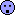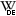BE-proff
●

2022-02-20 20:07
(589 d 21:34 ago)

Posting: # 22798
Views: 1,700

## Normal distribution assessment [General Sta­tis­tics]

Hi All,

One very general question that may be a little bit out of topicLet's say I have randomly generated set of 1 million values.What criterion should be used to check if the set has normal distribution?Helmut
★★★Vienna, Austria,
2022-02-20 23:59
(589 d 17:42 ago)

@ BE-proff
Posting: # 22799
Views: 1,415

## Normal distribution assessment

Hi BE-proff,

❝ Let's say I have randomly generated set of 1 million values.Before we apply a statistical method, we have to understand thedata generating process.
Therefore: How did you generate your data set? Obtained from RANDOM.ORG? With a hardware random number generator? Software? If yes, which PRNG? In the last case, most software (even Excel since 2010) implement the Mersenne Twis­ter, which is with its period of ≈4.3×106,001 fine for generating large data sets. However, in VBA still an LCG is im­ple­mented, which is bad for large data sets due to its shorter period.

❝ What criterion should be used to check if the set has normal distribution?Look at the histogram first.set.seed(123456)                                  # for reproducibility x   <- rnorm(1e6, mean = 0, sd = 1)               # or your data instead lim <- c(-max(abs(range(x))), max(abs(range(x)))) # for the plots hist(x, breaks = "FD", freq = FALSE, xlim = lim, col = "bisque", border = NA, las = 1) rug(x, side = 1, ticksize = 0.02) legend("topright", x.intersp = 0,        legend = c(paste("mean(x) =", signif(mean(x), 6)),                   paste("sd(x) =", signif(sd(x), 6))))

Does it look normal? Happy with the mean (should be ≈0) and the standard deviation (should be ≈1)?
If in doubt, overlay it with a kernel density estimate.

lines(density(x, n = 2^10), lwd = 3, col = "#FF000080")

Does it match? Not sure? Overlay the normal distribution.

curve(dnorm, lim, lim, n = 2^10, lwd = 2, col = "#0000FF80", add = TRUE)

Still in doubt?

plot(lim, lim, type = "n", xlab = "Theoretical Quantiles",      ylab = "Sample Quantiles", main = "Normal Q-Q Plot", las = 1) grid() qq <- qqnorm(x, plot.it = FALSE) points(qq$x, qq$y, pch = 21, cex = 1.25, col = "#87CEFA80", bg = "#87CEFA80") # patience... qqline(x)

If you insist in a test comparing the data’s empirical cumulative distribution function to the cumulative distribution function of the standard normal:

ks.test(x, "pnorm" , alternative = "two.sided")

Dif-tor heh smusma 🖖🏼 Довге життя Україна!Helmut SchützThe quality of responses received is directly proportional to the quality of the question asked. 🚮
Science Quotes
BE-proff
●

2022-02-21 15:42
(589 d 01:59 ago)

@ Helmut
Posting: # 22800
Views: 1,280

## Normal distribution assessment

Привет Helmut,

Many thanks for clarificationHelmut
★★★Vienna, Austria,
2022-02-22 16:11
(588 d 01:29 ago)

@ BE-proff
Posting: # 22801
Views: 1,279

## Testing ‘randomness’

Hi BE-proff,

❝ Many thanks for clarificationWelcome.

runs <- 25L n    <- 1e6 res  <- data.frame(run = 1:runs, p = NA_real_, sign = "") for (j in 1:nrow(res)) {   if (j == 1) set.seed(123456) else set.seed(j)   res$p[j] <- ks.test(rnorm(n = n), "pnorm", alternative = "two.sided")$p.value   if (res$p[j] < 0.05) res$sign[j] <- "*" } print(res, row.names = FALSE)

Dif-tor heh smusma 🖖🏼 Довге життя Україна!Helmut SchützThe quality of responses received is directly proportional to the quality of the question asked. 🚮
Science Quotes
Obinoscopy
★

USA,
2022-02-27 19:49
(582 d 21:52 ago)

@ BE-proff
Posting: # 22811
Views: 1,165

## Normal distribution assessment

❝ Let's say I have randomly generated set of 1 million values.❝ What criterion should be used to check if the set has normal distribution?I don't think a randomly generated set of numbers would have a normal distribution. Every number should have an equal chance of been selected so I would say it should look more like a uniform distribution. But that is just my thinking....

ScopyIng. Helmut Schütz